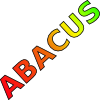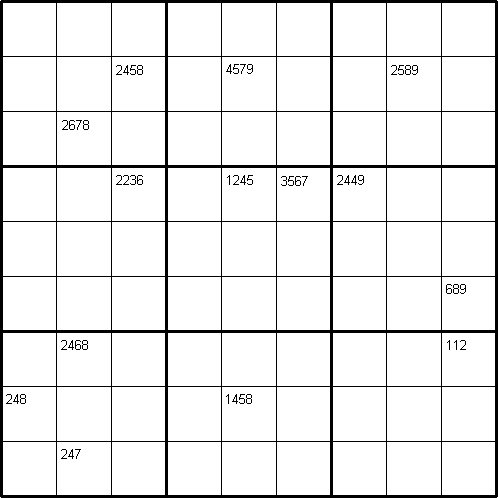#### You may also like### Consecutive Numbers

An investigation involving adding and subtracting sets of consecutive numbers. Lots to find out, lots to explore.### Tea Cups

Place the 16 different combinations of cup/saucer in this 4 by 4 arrangement so that no row or column contains more than one cup or saucer of the same colour.### Counting on Letters

The letters of the word ABACUS have been arranged in the shape of a triangle. How many different ways can you find to read the word ABACUS from this triangular pattern?

# Intersection Sudoku 2

##### Age 11 to 16Challenge Level
by Henry Kwok#### The Rules of Intersection Sudoku

Like the standard sudoku, this sudoku variant consists of a grid of nine rows and nine columns subdivided into nine 3x3 subgrids.
It has two basic rules:
• Each column, each row, and each box (3x3 subgrid) must have the numbers 1 through 9.
• No column, row or box can have two squares with the same number.
The puzzle can be solved with the help of small digits in the top parts of certain squares.

The small digits stand for the numbers in the squares horizontally and vertically adjacent to the square that contains the set of small digits. The number of small digits in each set can range from a minimum of 2 (in a corner of the puzzle) to a maximum of 4 (in any square beyond the edge of the puzzle).

#### A Short Demonstration

The square (3, 6) and the square (5, 6) of this puzzle contain a set of four small digits {2, 2, 3, 6} and {1, 2, 4, 5} respectively. If we colour the squares vertically and horizontally adjacent to (3, 6) with blue, and the squares vertically and horizontally adjacent to (5, 6) with yellow, we find that the square (4, 6) is coloured both with blue and yellow. It is the intersection of two sets of coloured squares, hence the name "Intersection Sudoku".